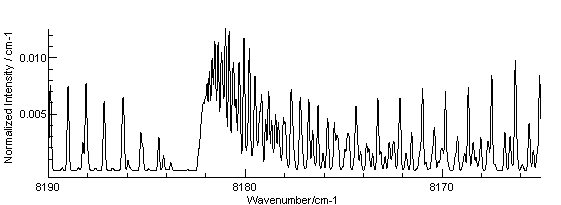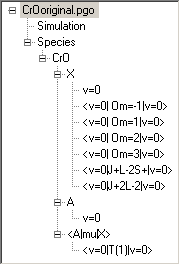Molecule Types Linear Molecules Samples

# Electronic spectrum of the A5Σ - X5Π transition of CrOThis example is based on A. S-C. Cheung, W. Zyrnicki and A. J. Merer, J. Molec. Spectrosc. 104 315 (1984), and shows an example of a  spectrum involving states with high multiplicity, and also demonstrates two alternative approaches to dealing with molecular parameters that are not presented in quite the same way as PGOPHER requires them. Five slightly different data files are given below. The difference between them is not really significant on the scale of the precision of the original data, but CrOAdjusted.pgo is the recommended one to use.

CrOoriginal.pgo. This gives the data in a form that is closest to the form presented in the paper, which requires special consideration as follows:

• The RSquaredH parameter is set to true, as the Hamiltonian specified in the paper is closest to this form.
• LimitSearch is set to true to give quantum number assignments consistent with the paper.
•The origins of the individual components of the ground X5Π state are specified individually, rather than in several spin orbit coupling constants. This effect can be achieved in PGOPHER using perturbations, in this case homogeneous contributions with OmegaSelect set. For example <v=0|Om=3|v=0> shifts the Ω = 3 component (only) by the amount of the perturbation Value. For correct assignment of the quantum numbers PGOPHER needs to know whether the state is regular or inverted. One approach is to set an approximately correct value for the spin-orbit coupling constant, A. in which case not all the components can be positioned in this way. For the file given here, the Ω = 0 and 1 components are positioned by setting the state Origin and A values, and perturbations are used to position the other spin-orbit components. In this case it is possible to derive the values by comparing the matrix elements used in PGOPHER and the paper, though in general it can be simpler to simply re-fit data from the literature.
As an example of how this can be done, consider the diagonal Ω = 0 matrix elements given in the paper for the X5Π state (Table I):
`T0+ (B-AD-2LambdaD+/-3*oD)*(J*(J+1)+5)- D*(J*J*(J+1)*(J+1)+20*J*(J+1)+25)+/-3*(o+p+q)`

PGOPHER gives the following for the same matrix elements (right click on X and select matrix elements):

`<J,Lambda=1,Omega=0 + |H| + J,Lambda=1,Omega=0> = +  Origin+  B*(J*(J+1)+5)+  -A+  + 3*o+  -6*gamma+  D*(-J*(J*(J*(J+2)+21)+20)-25)+  (AD+2*LambdaD)*(-J*(J+1)-5)+  +3*oD*(J*(J+1)+5)`

A little algebra will show that these two expressions are identical apart from o and oD (discussed below) and the J independent terms. Equating these shows that  TO = Origin-A-6*gamma. Repeating the process with Ω = 1 shows that T1 = Origin-6*gamma. The difference between these, T1 - T0 =  A, so given that the paper fixes T0 = 0 it follows that A can be set to T1. The paper has chosen to take the energy zero for the state at Ω = 0, while PGOPHER will take it at Σ = 0 component (Ω = 1 here). To align the two scales, set the PGOPHER Origin = A+6*gamma. Repeating the process for the other Ω components gives the following set of equations:

`T(-1) = Origin-2*A-4*gamma+<v=0|Om=-1|v=0>T2 = Origin+A-4*gamma+<v=0|Om=2|v=0>T3 = Origin+2*A+<v=0|Om=3|v=0>`

Note that the homogeneous perturbations such as <v=0|Om=3|v=0> have been added here, and each of these is essentially indicating how far the component has been shifted from the equal spacing predicted from the simple spin orbit energy formula AΛΣ. The required value for each component can be worked out by rearranging the above giving, for example, <v=0|Om=2|v=0> =  T2-T0 - (2A+2*gamma).

• The lambda doubling constants are given in an alternative form that naturally involves J rather than N (see Λ doubling for more on this). The term involving o (in PGOPHER) has the same operator form as o+p+q as used in the paper, so no extra term is required for this. This also applies to its centrifugal distortion, oD, as confirmed by comparing the matrix elements above. To reproduce the p+2q term requires a perturbation, <v=0|J+L-2S+|v=0>; this uses Op = Luncouple, n = 2, Srank = -1 and Scomp = -2. To match the definition given in the paper with the PGOPHER perturbation requires a scale factor of -1/2; this can be worked out by comparing the operator forms (see perturbations for the PGOPHER definitions) or by comparing matrix elements. Similarly the <v=0|J+2L-2|v=0> perturbation is used to reproduce the q term; this time the scale factor is +1/2.
CrOoriginalAlternate.pgo. Thus file uses an alternate method of positioning the Ω components by setting A to zero, and using OmegaOrder set to Regular to force the correct state ordering. This was essentially generated from the file above by setting A and the origin to zero, and adding A(Ω-1) to all the J independent perturbations, though for exact agreement the origin needs to be set to .0912 = 6γ.
CrOoriginalAdjusted.pgo. This is the original data file, with some minor adjustments to give slightly better agreement with the energy levels and transitions listed in the paper. To do this, the information in table VIII and the appendix was converted to a form PGOPHER could use. Using the simplified format the energy level list can be input as follows:
`FrequencyOffset -54.43912NQN 2 - - 1 F1f 0.0002 - - 2 F1f 2.025 - - 3 F1f 5.0621 - - 4 F1f 9.1117 - - 14 F1f 105.3185 - - 15 F1f 120.5138 - - 0 F2f 57.1558 - - 1 F2f 58.1881 - - 0 F2e 56.9666 - - 1 F2e 58.0008 - - 2 F2e 60.0692 - - 3 F2e 63.1718`

Only a few entries are shown above; the complete file is available as CrOTableVIII.lin. Notes on producing this:

• The e and f levels have been swapped, to give energy levels consistent with the signs of the published lambda doubling constants, which were in turn chosen on the assumption that the upper state is a 5Σ - state. The true sign, and thus the absolute parity assignments, was not known and the tabulated energy levels seem to have been worked out with the opposite signs of the constants. In general swapping + and - parity assignments, and swapping Σ - and Σ + states will make no change to the spectrum, provided the signs of all the lambda doubling constants are also changed.
• The FrequencyOffset directive is required as the energy zero for this list is taken as the lowest energy level, J = 1 of the Ω = -1 component.

Given the settings above, table VIII can be reproduced exactly if minor adjustments by fitting are made to the Origin, B, o, gamma and the perturbations. The adjustments are mainly because the constants have not been quoted to sufficient precision to reproduce the calculated values.

The transitions given in the appendix can be similarly converted into a form that PGOPHER can read using the BranchTable format:

`BranchTable sR54 rQ54  qP54    pQ34      oQ24     oP34     nP247  8021.3   8011.337    -        -        -        -        -    8  8023.748 8012.779 8002.799    -     7988.457    -        -    9  8026.122 8014.157 8003.182    -     7986.855    -        -    10 8028.406 8015.457 8003.513    -        -        -     7976.19411 8030.687 8016.698 8003.742    -     7983.441    -     7973.45112 8032.814 8017.876 8003.909    -     7981.63     -     7970.64913 8034.913 8018.965 8004.007    -     7979.758    -     7967.77814 8036.943 8020.003 8004.04  7992.332 7977.818    -     7964.82215 8038.898 8020.967 8004.007 7991.327 7975.811 7976.352 7961.85216 8040.787 8021.867 8003.909 7990.252 7973.74  7974.309 7958.792`

Again, only a few sample lines are shown; the full list is available as CrOAppendix.lin. The agreement with the observed lines is good, but can be slightly improved (average error dropping from 0.011 to 0.0086 cm-1) by fitting the upper state Origin, B and LambdaD. The changes are minor, with the exception of LambdaD which changes from 4.6e-6 to 1.6e-6. This may reflect a misprint in the original paper, or possibly a more thorough treatment of blended transitions in the original fit.

CrO.pgo is set up using the standard constants available in PGOPHER, derived as follows:

• The RSquaredH parameter left at false, the default.
• LimitSearch is set to true to give quantum number assignments consistent with the paper.
• The Origin for the ground X5Π state is left at zero, the default, and values for A, LambdaSS and eta are taken from equation (20) of the paper.
• The lambda doubling constants o, p and q are worked out from the combined values given in Table V
• The Origin of the upper A5Σ - state needs to be offset by approximately the gap between the Ω = 0 and Ω = 1; the exact value is taken to give the best fit to the tabulated values. This arises from the different choice of energy zero for the ground state used by PGOPHER.
CrOAdjusted.pgo is the same data file, with selected constants adjusted slightly to give the best fit with the energy levels and transitions values given in the paper.
• For the ground state table VIII can be reproduced exactly if minor adjustments by fitting are made to the Origin, B, A, LambdaSS, eta, o, theta, AD and eta of the ground state. The adjustments are mainly because the constants have not been quoted to sufficient precision to reproduce the calculated values, and minor differences to the matrix elements. Note that fitting requires a FrequencyOffset of -119.72578 as the choice of energy zero is different.
• For the excited state Origin, B and LambdaD are fitted. The changes are minor, with the exception of LambdaD which changes from 4.6e-6 to 1.6e-6. This may reflect a misprint in the original paper, or including blended transitions in the fit. The average error drops from 0.011 to 0.0086 cm-1 on fitting.